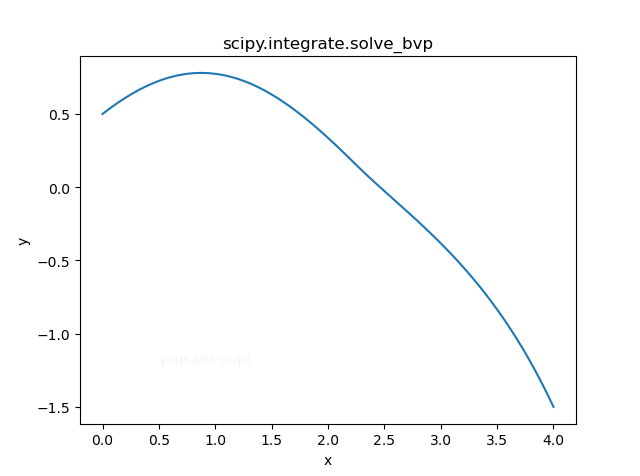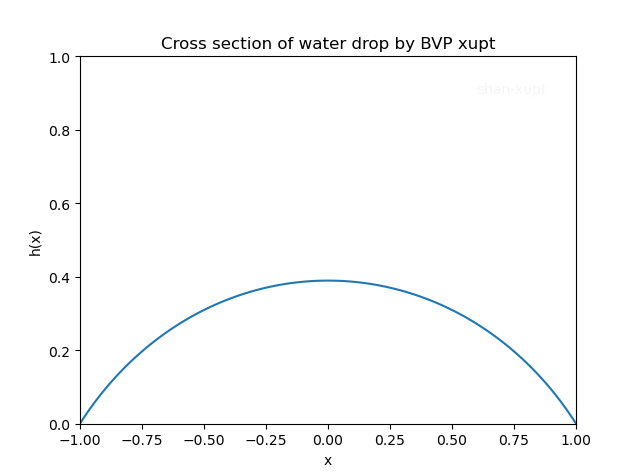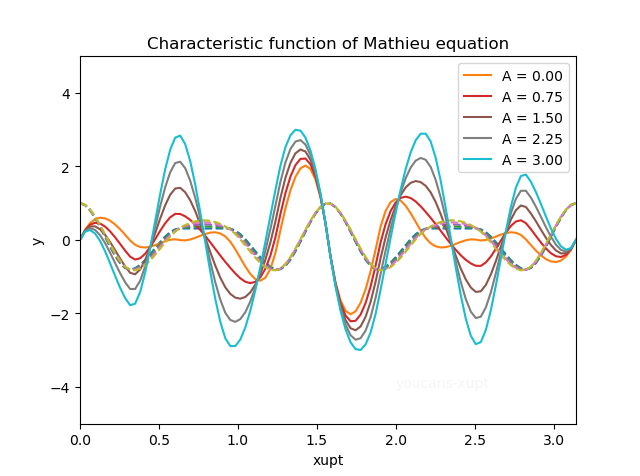# Python小白的数学建模课-10.微分方程边值问题

## 1. 常微分方程的边值问题（BVP）

### 1.2 常微分方程边值问题的数学模型

$y{\ ''} = f(x,y,y{\ '})，\; a<x<b$

（1）第一类边界条件（两点边值问题）：

$y(a)=ya，y(b)=yb$

（2）第二类边界条件：

$y\ '(a)=ya，y\ '(b)=yb$

（3）第三类边界条件：

$\begin{cases} y\ '(a)-a_0\ y(a) = a_1\\ y\ '(b)-b_0\ y(b) = b_1 \end{cases}$

## 2. SciPy 求解常微分方程边值问题

### 2.1 BVP 问题的标准形式

Scipy 用 solve_bvp() 函数求解常微分方程的边值问题，定义微分方程的标准形式为：

$\begin{cases} y{\ '} = f(x,y)，\; a<x<b\\ g(y(a),y(b)=0) \end{cases}$

$\begin{cases} y(a)-ya=0\\ y(b)-yb=0 \end{cases}$

### 2.2 scipy.integrate.solve_bvp() 函数

**scipy.integrate.solve_bvp() **是求解微分方程边值问题的具体方法，可以求解一阶微分方程（组）的两点边值问题（第一类边界条件）。在 odeint 函数内部使用 FORTRAN 库 odepack 中的 lsoda，可以求解一阶刚性系统和非刚性系统的初值问题。官网介绍详见： scipy.integrate.solve_bvp — SciPy v1.7.0 Manual

scipy.integrate.solve_bvp(fun, bc, x, y, p=None, S=None, fun_jac=None, bc_jac=None, tol=0.001, max_nodes=1000, verbose=0, bc_tol=None)


solve_bvp 的主要参数：

• func: callable fun(x, y, ..) 　　导数函数 $f(y,x)$ ， y 在 x 处的导数，以函数的形式表示。可以带有参数 p。
• bc: callable bc(ya, yb, ..) 　　边界条件，y 在两点边界的函数，以函数的形式表示。可以带有参数 p。
• x: array：　　初始网格的序列，shape (m,)。必须是单调递增的实数序列，起止于两点边界值 xa，xb。
• y: array：　　网格节点处函数值的初值，shape (n,m)，第 i 列对应于 x[i]。
• p: array：　　可选项，向导数函数 func、边界条件函数 bc 传递参数。

solve_bvp 的主要返回值：

• sol: PPoly 　　通过 PPoly （如三次连续样条函数）插值求出网格节点处的 y 值。
• x: array 　　数组，形状为 (m,)，最终输出的网格节点。
• y: array 　　二维数组，形状为 (n,m)，输出的网格节点处的 y 值。
• yp: array 　　二维数组，形状为 (n,m)，输出的网格节点处的 y' 值。

## 3. 实例 1：一阶常微分方程边值问题

### 3.1 例题 1：一阶常微分方程边值问题

\begin{cases} \begin{aligned} &y\ ''+ \left| y \right| = 0\\ &y(x=0) = 0.5\\ &y(x=4) = -1.5 \end{aligned} \end{cases}

$\begin{cases} y_0^{'} = y_1\\ y_1^{'} = -\left| y_0 \right| \\ y(x=0) - 0.5 = 0\\ y(x=4) + 1.5 = 0 \end{cases}$

### 3.2 常微分方程的编程步骤

1. 导入 scipy、numpy、matplotlib 包；

2. 定义导数函数 dydx(x,y)

注意本问题中 y 表示向量，记为 $y=[y_0,y_1]$，导数定义函数 dydx(x,y) 编程如下：

# 导数函数，计算导数 dY/dx
def dydx(x, y):
dy0 = y
dy1 = -abs(y)
return np.vstack((dy0, dy1))

1. 定义边界条件函数 boundCond(ya,yb)

本问题中边界条件为常数，具体编程如下。注意对照 3.1中的边值条件，没有为什么，函数就是这么定义的。

# 计算 边界条件
def boundCond(ya, yb):
fa = 0.5  # 边界条件 y(xa=0) = 0.5
fb = -1.5  # 边界条件 y(xb=4) = -1.5
return np.array([ya-fa,yb-fb])

1. 设置 x、y 的初值

2. 调用 solve_bvp() 求解常微分方程在区间 [xa,xb] 的数值解

3. 由 solve_bvp() 的返回值 sol，获得网格节点的处的 y值。

### 3.3 Python 例程

# mathmodel11_v1.py
# Demo10 of mathematical modeling algorithm
# Solving ordinary differential equations (boundary value problem) with scipy.

from scipy.integrate import odeint, solve_bvp
import numpy as np
import matplotlib.pyplot as plt

# 1. 求解微分方程组边值问题，DEMO
# y'' + abs(y) = 0, y(0)=0.5, y(4)=-1.5

# 导数函数，计算导数 dY/dx
def dydx(x, y):
dy0 = y
dy1 = -abs(y)
return np.vstack((dy0, dy1))

# 计算 边界条件
def boundCond(ya, yb):
fa = 0.5  # 边界条件 y(xa=0) = 0.5
fb = -1.5  # 边界条件 y(xb=4) = -1.5
return np.array([ya-fa,yb-fb])

xa, xb = 0, 4  # 边界点 (xa,xb)
# fa, fb = 0.5, -1.5  # 边界点的 y值
xini = np.linspace(xa, xb, 11)  # 确定 x 的初值
yini = np.zeros((2, xini.size))  # 确定 y 的初值
res = solve_bvp(dydx, boundCond, xini, yini)  # 求解 BVP

xSol = np.linspace(xa, xb, 100)  # 输出的网格节点
ySol = res.sol(xSol)  # 网格节点处的 y 值

plt.plot(xSol, ySol, label='y')
# plt.legend()
plt.xlabel("x")
plt.ylabel("y")
plt.title("scipy.integrate.solve_bvp")
plt.show()


### 3.4 Python 例程运行结果## 4. 实例 2：水滴横截面的形状

### 4.1 例题 2：水滴横截面形状问题

\begin{cases} \begin{aligned} &\frac{d^2 h}{dx^2} + [1-h]*[1+(\frac{dh}{dx})^2]^{3/2}= 0\\ &h(x=-1) = h(x=1) = 0 \end{aligned} \end{cases}

$\begin{cases} h_0^{'} = h_1\\ h_1^{'} = (h_0-1) * [1+h_1^2]^{3/2}\\ h_0(x=-1) = h_0(x=1) = 0 \end{cases}$

### 4.2 Python 例程：水滴横截面形状问题

# mathmodel11_v1.py
# Demo10 of mathematical modeling algorithm
# Solving ordinary differential equations (boundary value problem) with scipy.

from scipy.integrate import odeint, solve_bvp
import numpy as np
import matplotlib.pyplot as plt

# 3. 求解微分方程边值问题，水滴的横截面
# 导数函数，计算 h=[h0,h1] 点的导数 dh/dx
def dhdx(x,h):
# 计算 dh0/dx, dh1/dx 的值
dh0 = h  # 计算 dh0/dx
dh1 = (h-1)*(1+h*h)**1.5  # 计算 dh1/dx
return np.vstack((dh0, dh1))

# 计算 边界条件
def boundCond(ha,hb):
# ha = 0  # 边界条件：h0(x=-1) = 0
# hb = 0  # 边界条件：h0(x=1) = 0
return np.array([ha,hb])

xa, xb = -1, 1  # 边界点 (xa=0, xb=1)
xini = np.linspace(xa, xb, 11)  # 设置 x 的初值
hini = np.zeros((2, xini.size))  # 设置 h 的初值

res = solve_bvp(dhdx, boundCond, xini, hini)   # 求解 BVP
# scipy.integrate.solve_bvp(fun, bc, x, y,..)
#   fun(x, y, ..), 导数函数 f(y,x)，y在 x 处的导数。
#   bc(ya, yb, ..), 边界条件，y 在两点边界的函数。
#   x: shape (m)，初始网格的序列，起止于两点边界值 xa，xb。
#   y: shape (n,m)，网格节点处函数值的初值，第 i 列对应于 x[i]。

xSol = np.linspace(xa, xb, 100)  # 输出的网格节点
hSol = res.sol(xSol)  # 网格节点处的 h 值
plt.plot(xSol, hSol, label='h(x)')
plt.xlabel("x")
plt.ylabel("h(x)")
plt.axis([-1, 1, 0, 1])
plt.title("Cross section of water drop by BVP xupt")
plt.show()


### 4.3 Python 例程运行结果## 5. 实例 3：带有未知参数的微分方程边值问题

### 5.1 例题 3：Mathieu 方程的特征函数

Mathieu 在研究椭圆形膜的边界值问题时，导出了一个二阶常微分方程，其形式为：

$\frac{d^2 y}{dx^2} + [\lambda-2q\ cos(2x)]\ y= 0$

$\begin{cases} y_0^{'} = y_1\\ y_1^{'} = -[\lambda-2q\ cos(2x)]\ y_0 \\ y_0(x=0) = 1\\ y_1(x=0) = 0\\ y_1(x=\pi)=0 \end{cases}$

### 5.2 常微分方程的编程步骤

1. 导入 scipy、numpy、matplotlib 包；

2. 定义导数函数 dydx(x,y,p)

本问题中 y 表示向量，记为 $y=[y_0,y_1]$，定义函数 dydx(x,y,p) 中的 p 是待定参数。

# 导数函数，计算导数 dY/dx
def dydx(x, y, p): # p 是待定参数
lam = p  # 待定参数，从 solve-bvp() 传递过来
q = 10  # 设置参数
dy0 = y
dy1 = -(lam-2*q*np.cos(2*x))*y
return np.vstack((dy0, dy1))

1. 定义边界条件函数 boundCond(ya,yb,p)

注意，虽然边界条件定义函数并没有用到参数 p，但也必须写在输入变量中，函数就是这么要求的。

# 计算 边界条件
def boundCond(ya, yb, p):
lam = p
return np.array([ya-1,ya,yb])

1. 设置 x、y 的初值

2. 调用 solve_bvp() 求解常微分方程在区间 [xa,xb] 的数值解

3. 由 solve_bvp() 的返回值 sol，获得网格节点的处的 y值。

### 5.3 Python 例程

# mathmodel11_v1.py
# Demo10 of mathematical modeling algorithm
# Solving ordinary differential equations (boundary value problem) with scipy.

from scipy.integrate import odeint, solve_bvp
import numpy as np
import matplotlib.pyplot as plt

# 4. 求解微分方程组边值问题，Mathieu 方程
# y0' = y1, y1' = -(lam-2*q*cos(2x))y0)
# y0(0)=1, y1(0)=0, y1(pi)=0

# 导数函数，计算导数 dY/dx
def dydx(x, y, p): # p 是待定参数
lam = p
q = 10
dy0 = y
dy1 = -(lam-2*q*np.cos(2*x))*y
return np.vstack((dy0, dy1))

# 计算 边界条件
def boundCond(ya, yb, p):
lam = p
return np.array([ya-1,ya,yb])

xa, xb = 0, np.pi  # 边界点 (xa,xb)
xini = np.linspace(xa, xb, 11)  # 确定 x 的初值
xSol = np.linspace(xa, xb, 100)  # 输出的网格节点

for k in range(5):
A = 0.75*k
y0ini = np.cos(8*xini)  # 设置 y0 的初值
y1ini = -A*np.sin(8*xini)  # 设置 y1 的初值
yini = np.vstack((y0ini, y1ini))  # 确定 y=[y0,y1] 的初值
res = solve_bvp(dydx, boundCond, xini, yini, p=)  # 求解 BVP
y0 = res.sol(xSol)  # 网格节点处的 y 值
y1 = res.sol(xSol)  # 网格节点处的 y 值
plt.plot(xSol, y0, '--')
plt.plot(xSol, y1,'-',label='A = {:.2f}'.format(A))

plt.xlabel("xupt")
plt.ylabel("y")
plt.title("Characteristic function of Mathieu equation")
plt.axis([0, np.pi, -5, 5])
plt.legend(loc='best')
plt.text(2,-4,"youcans-xupt",color='whitesmoke')
plt.show()


### 5.4 Python 例程运行结果## 6. 小结

1. 微分方程的边值问题相对初值问题来说更为复杂，但是用 Scipy 工具包求解标准形式的微分方程边值问题，编程实现还是不难掌握的。
2. 关于边值问题的模型稳定性、灵敏度的分析，是更为专业的问题。除非找到专业课程教材或范文中有相关内容可以参考套用，否则不建议小白自己摸索，这些问题不是调整参数试试就能试出来的。

【本节完】